##### Trigonometry For DummiesA 45 – 45 – 90 degree triangle (or isosceles right triangle) is a triangle with angles of 45°, 45°, and 90° and sides in the ratio of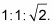Note that it’s the shape of half a square, cut along the square’s diagonal, and that it’s also an isosceles triangle (both legs have the same length). The following figure shows an example of a 45°- 45°- 90° triangle.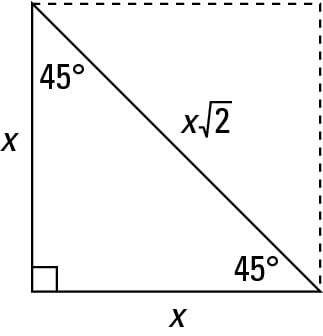Try a couple of problems. Find the lengths of the unknown sides in triangles BAT and BOY shown in the following figure.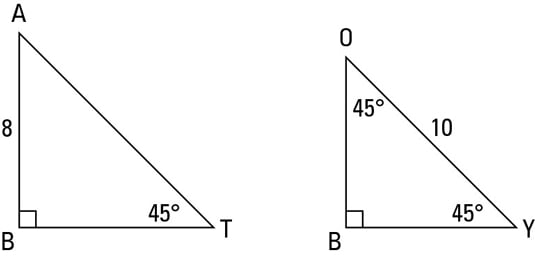You can solve 45°- 45°- 90° triangle problems in two ways: the formal book method and the street-smart method. Try ’em both and take your pick.

## Using the formal book method

The formal method uses the ratio of the sides from the first figure.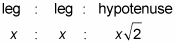For triangle BAT, because one of the legs is 8, the x in the ratio is 8. Plugging 8 into the three x’s gives you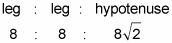And for triangle BOY, the hypotenuse is 10, so you set the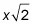from the ratio equal to 10 and solve for x: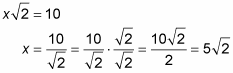That does it: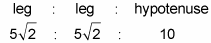## Using the street-smart method

Now for the street-smart method for working with the 45°- 45°- 90° triangle (this method is based on the same math as the formal method, but it involves fewer steps). Remember the 45°- 45°- 90° triangle as the “root two triangle.” Using that tidbit, do one of the following: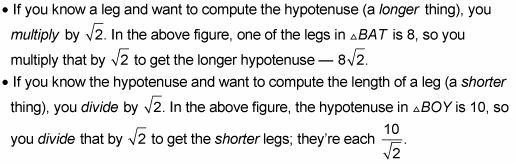Look back at the lengths of the sides in triangle BAT and triangle BOY. In triangle BAT, the hypotenuse is the only side that contains a radical. In triangle BOY, the hypotenuse is the only side without a radical. These two cases are by far the most common ones, but in unusual cases, all three sides may contain a radical symbol.